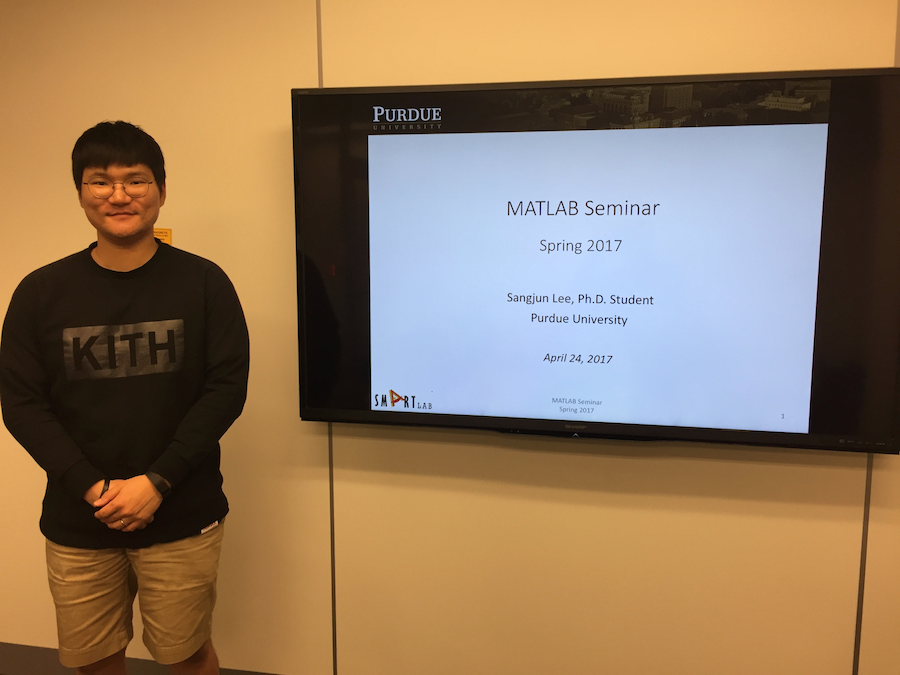### Intorduction to MATLAB Programming By Sangjun Lee

Spring 2017

MATLAB is a powerful research software tool and it has been widely employed to scientific simulation and experiment. This weekly workshop will give students fluency in MATLAB, including popular toolboxes such as robotics and optimization toolboxes. The workshop consists of interactive lectures with students doing sample MATLAB problems in real time. At the end of the workshop, students will be able to use MATLAB in their own work.

The following is the seminar schedule:
Week 1: Introduction to MATLAB programming
Week 2: Import data from a real sensor measurement and generate plots
Week 3: Open and parse rosbag log file